### The Working Basis Matrix

Let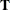be the basis matrix of NPSC. The following partitioning is done: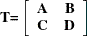where

•is the number of nodes.

•is the number of side constraints.

•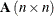is the network component of the basis. Most of the columns of this matrix are columns of the problem’s node-arc incidence matrix. The arcs associated with columns of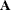, called key basic variables or key arcs, form a spanning tree. The data structures of the spanning tree of this submatrix of the basisenable the computations involvingand the manner in whichis updated to be very efficient, especially those dealing with(or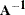).

•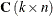are the key arcs’ side constraint coefficient columns.

•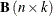are the node-arc incidence matrix columns of the nontree arcs. The columns of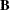having nonzero elements are associated with basic nonspanning tree arcs.

•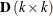are the constraint coefficient columns of nonkey basic variables. Nonkey basic variables not only include nontree basic arcs but also basic slack, surplus, artificial, or nonarc variables.

It is more convenient to factorby block triangular matrices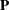and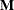, such that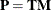. The matricesandare used instead ofbecause they are less burdensome to work with. You can perform block substitution when solving the simplex iteration linear systems of equations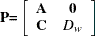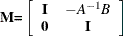where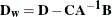and is called the working basis matrix.

To perform block substitution, you need the tree data structure of thematrix, and also the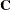,, and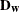matrices. Because thematrix consists of columns of the constraint coefficient matrix, the maintenance offrom iteration to iteration simply entails changing information specifying which columns of the constraint coefficient matrix compose.

The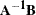matrix is usually very sparse. Fortunately, the information incan be initialized easily using the tree structures. In most iterations, only one column is replaced by a new one. The values of the elements of the new column may already be known from preceding steps of the simplex iteration.

The working basis matrix is the submatrix that presents the most computational complexity. However, PROC NETFLOW usually can use classical simplex pivot techniques. In many iterations, only one column ofchanges. Sometimes it is not necessary to updateor its inverse at all.

If INVD_2D is specified in the PROC NETFLOW statement, only one row and one column may need to be changed in the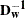before the next simplex iteration can begin. The new contents of the changed column are already known. The new elements of the row that changes are influenced by the contents of a row ofthat is very sparse.

If INVD_2D is not specified in the PROC NETFLOW statement, the Bartels-Golub update can be used to update the LU factors of. The choice must be made whether to perform a series of updates (how many depends on the number of nonzeros in a row of), or refactorization.